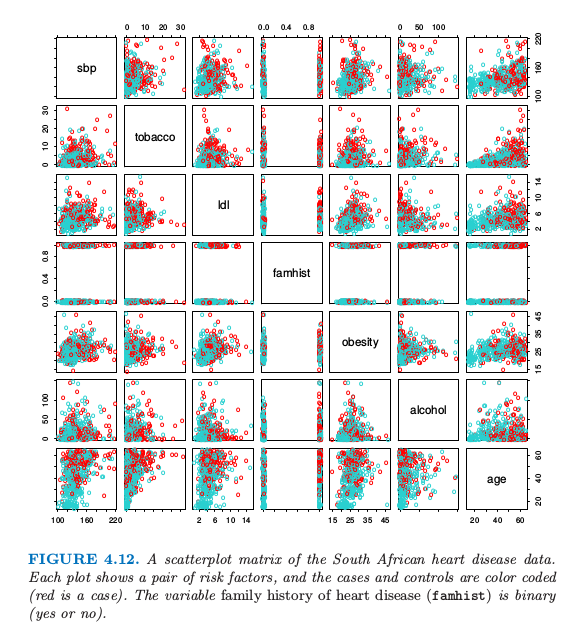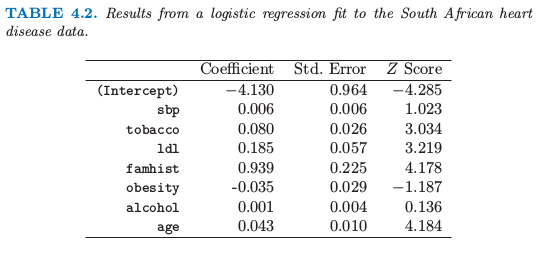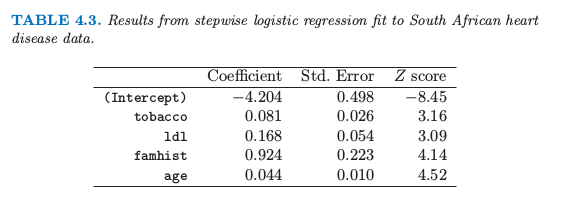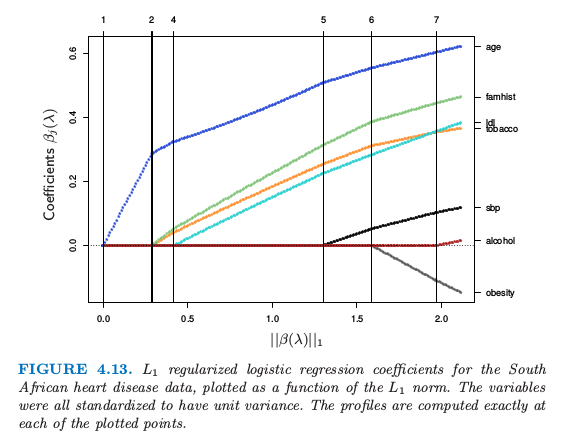4.4 逻辑斯蒂回归¶

weiya 注:

拟合逻辑斯蒂回归模型¶

weiya 注：多项式分布的合理性

$N$ 个观测的对数似然为

weiya 注

Newton-Raphson 也就是我们熟知的牛顿迭代法

weiya 注

$\mathbf X=[x’_1,x’_2,\ldots,x’_N]’$

weiya注：IRLS

Github: xqwen/IRLS用C++实现IRLS．

weiya 注：

例子：南非心脏病¶二次拟合和推断¶

• 加权的残差平方和是熟悉的 Pearson 卡方统计量 这是对偏差的二次近似．
• 极限概率理论表明如果模型是正确的，则 $\hat\beta$ 为常数（即收缩到真实的 $\beta$）
• 中心极限定理证明 $\hat \beta$ 的分布收敛到 $N(\beta,\mathbf{(X^TWX)^{-1}})$．这个可以模仿正态理论推断直接从加权最小二乘得到．
• 对于逻辑斯蒂回归建立模型计算量比较大，因为每个模型拟合需要迭代．受欢迎的方法是 Rao score 检验来检验是否包含某一项，以及 Wald 检验来检验是否剔除某一项．这些都不需要迭代拟合，而且都是基于当前模型的极大似然拟合．结果是两者都代表在加权最小二乘拟合中运用相同的系数加上或剔除某一项．这些计算可以高效地进行，不需要重新计算整个加权最小二乘拟合．

$L_1$正则化逻辑斯蒂回归¶

Lasso 中使用的 $L_1$ 惩罚（3.4.2节）可以应用到任意线性回归模型的变量选择和收缩上面．对于逻辑斯蒂回归，我们最大化 \eqref{4.20} 带惩罚项的版本：

weiya 注: Recall逻辑斯蒂回归或者 LDA？¶

Info

4.3 节我们发现类别 $k$ 和 $K$ 类关于 $x$ 线性函数的后验概率 odds 的对数 \eqref{4.9}：

weiya 注: Recall

1. Friedman, J., Hastie, T. and Tibshirani, R. (2010). Regularization paths for generalized linear models via coordinate descent, Journal of Statistical Software 33(1): 1–22.

2. Rousseauw, J., du Plessis, J., Benade, A., Jordaan, P., Kotze, J., Jooste, P. and Ferreira, J. (1983). Coronary risk factor screening in three rural communities, South African Medical Journal 64: 430–436.

3. Hastie, T. and Tibshirani, R. (1987). Nonparametric logistic and proportional odds regression, Applied Statistics 36: 260–276.

4. Park, M. Y. and Hastie, T. (2007). l1-regularization path algorithm for generalized linear models, Journal of the Royal Statistical Society Series B 69: 659–677.

5. Koh, K., Kim, S.-J. and Boyd, S. (2007). An interior-point method for large-scale L1-regularized logistic regression, Journal of Machine Learning Research 8: 1519–1555.

6. Efron, B. (1975). The efficiency of logistic regression compared to normal discriminant analysis, Journal of the American Statistical Association 70: 892–898.